Select Page

# Maths 12 Science CBSE Inverse Trigonometric Functions Answers for MCQ in English

Maths 12 Science CBSE Inverse Trigonometric Functions Answers for MCQ in English to enable students to get Answers in a narrative video format for the specific question.

Expert Teacher provides Maths 12 Science CBSE Inverse Trigonometric Functions Answers for MCQ through Video Answers in English language. This video solution will be useful for students to understand how to write an answer in exam in order to score more marks. This teacher uses a narrative style for a question from Inverse Trigonometric Functions not only to explain the proper method of answering question, but deriving right answer too.

Please find the question below and view the Answer in a narrative video format.

Question:

## Similar Questions from CBSE, 12th Science, Maths, Inverse Trigonometric Functions

Question 1 :

Write the value of the following: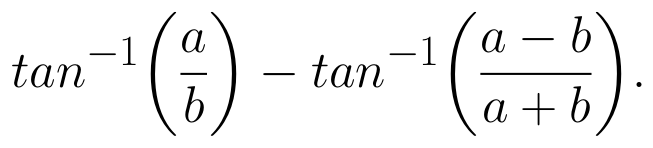Question 2 : Write the principal value of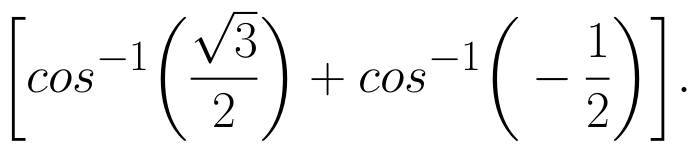(View Answer Video)

Question 3 :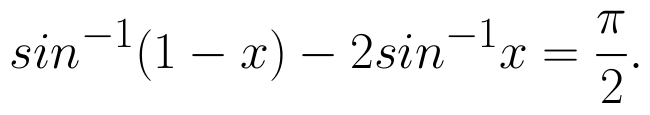Then x is equal to : (View Answer Video)

Question 4 :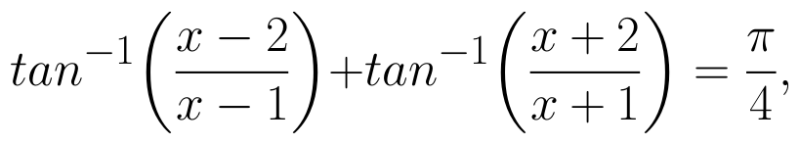then find the value of x. (View Answer Video)

Question 5 : Write in the simplest form: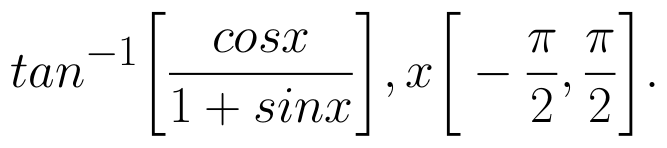(View Answer Video)

### Determinant

Question 1 : Let A be a square matrix of order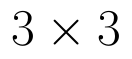, then |kA| is equal to, (View Answer Video)

Question 2 : If A is a symmetric matrix, then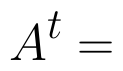? (View Answer Video)

Question 3 : Evaluate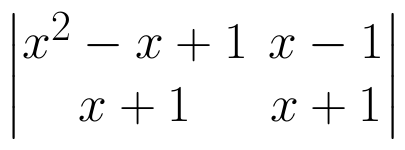. (View Answer Video)

Question 4 : Evaluate  the determinants in :.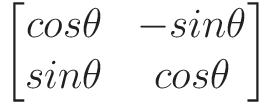(View Answer Video)

Question 5 : Evaluate the determinant: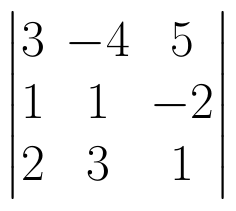. (View Answer Video)

### Integrals

Question 1 : Find: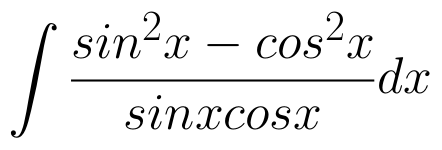Question 2 : Evaluate :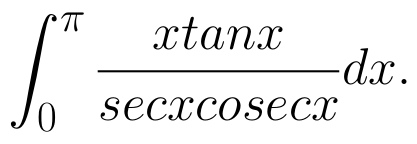(View Answer Video)

Question 3 : Write an antiderivative for the function,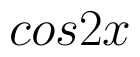using the method of inspection. (View Answer Video)

Question 4 : Find the integral of the function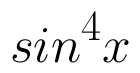. (View Answer Video)

Question 5 : Find :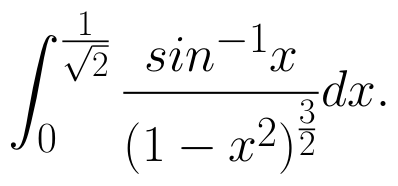(View Answer Video)

### Application of Integrals

Question 1 : Find the area of the smaller region bounded by the ellipse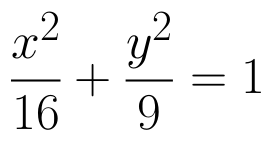and the straight line 8x + 3y = 12. (View Answer Video)

Question 2 : Find the area of the region bounded by the curves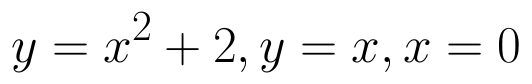and x = 3. (View Answer Video)

Question 3 : Find the area between the curves y = x and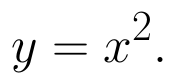(View Answer Video)

Question 4 : Using integration find the area of the triangular region whose sides have the equations y = 2x + 1, y = 3x + 1 and x = 4. (View Answer Video)

Question 5 : Using integration, find the area of the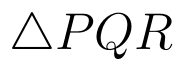co-ordinates whose vertices are P(2,0), Q(4, 5) and R(6,3). (View Answer Video)